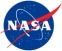# Grouping breaks cell formatting

GridTop achievementsRank 2
Erik asked on 30 Mar 2017, 02:36 PM

We are using code from this example Here in the databinding of our grid to conditionally set cell background color but when we turn on grouping, the code fails due to the addition of the grouping rows added.  we have tried getting the child rows of the group but just cannot get the syntax right.  Any help would be great.

Thanks,

Erik

`for` `(``var` `x = 0; x < Months.length; x++) {`
`    ``// get the index of the column`
`    ``var` `columnIndex = ``this``.wrapper.find(``".k-grid-header [data-field="` `+ Months[x] + ``"]"``).index()-1;`

`    ``// iterate the data items and apply row styles where necessary`
`    ``var` `dataItems = e.sender.dataSource.view();`
`    ``for` `(``var` `j = 0; j < dataItems.length; j++) {`
`        ``var` `group = e.sender.tbody.find(``".k-grouping-row"``);`
`        ``var` `row = group.children().find(``"[data-uid='"` `+ dataItems[j].uid + ``"']"``);`
`        ``var` `cell = row.children().eq(columnIndex);`
`        ``var` `monthNum = x + 1;`
`        ``if` `(x === 12) {`
`            ``monthNum = 12;`
`        ``}`
`        ``if` `(((selFY === curfyear) && (curfmon > monthNum)) || (selFY < curfyear)) {`
`            ``cell.addClass(``'disabledColor'``);`
`        ``}`
`    ``}`

## 2 Answers, 1 is accepted

0Viktor Tachev
Telerik teamanswered on 03 Apr 2017, 09:28 AM
Hi Erik,

Note that when grouping is applied the view() method for the DataSource would return the groups. In that case you can get the items with the data() method. The dataBound handler would look similar to the following.

`dataBound: ``function``(e) {`
`    ``// get the index of the UnitsInStock cell`
`    ``var ``columns = e.sender.columns;`
`    ``var ``columnIndex = ``this``.wrapper.find(``".k-grid-header [data-field=" ``+ ``"UnitsInStock" ``+ ``"]"``).index();`

`    ``// iterate the data items and apply row styles where necessary`
`    ``var ``dataItems = e.sender.dataSource.data();`
`    ``for ``(``var ``j = 0; j < dataItems.length; j++) {`
`        ``var ``units = dataItems[j].get(``"UnitsInStock"``);`
`        ``var ``discontinued = dataItems[j].get(``"Discontinued"``);`

`        ``var ``row = e.sender.tbody.find(``"[data-uid='" ``+ dataItems[j].uid + ``"']"``);`
`        ``if ``(discontinued) {`
`            ``row.addClass(``"discontinued"``);`
`        ``}`

`        ``var ``cell = row.children().eq(columnIndex);`
`        ``cell.addClass(getUnitsInStockClass(units));`
`    ``}`
`}`

For your convenience I have applied the approach in the dojo example. Check it out below and let me know how it works for you.

Regards,
Viktor Tachev
Telerik by Progress
Try our brand new, jQuery-free Angular 2 components built from ground-up which deliver the business app essential building blocks - a grid component, data visualization (charts) and form elements.
0Erik
Top achievementsRank 2
answered on 03 Apr 2017, 11:03 AM
This worked perfect, Thanks!
Tags
GridErik
Top achievementsRank 2Rank 2